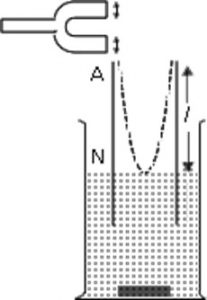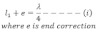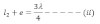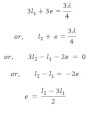# End Correction

In actual practice, antinode is not formed at the opened, but it is formed a little above from the open end. Then the difference between actual position of the antinode and the position f the open end is called end correction.According to the Rayleigh, “the end correction for the closed organ pipe is 0.3d and 0.6 d for the open organ pipe. Where d is the internal diameter of the pipe.”
If to be the first resonating length and be the wave length of the wave in the pipe then in first resonation,Similarly if l2 be the second resonating length and be the wavelength of wave in the pipe, then,Solving equation (i) and (ii), we get